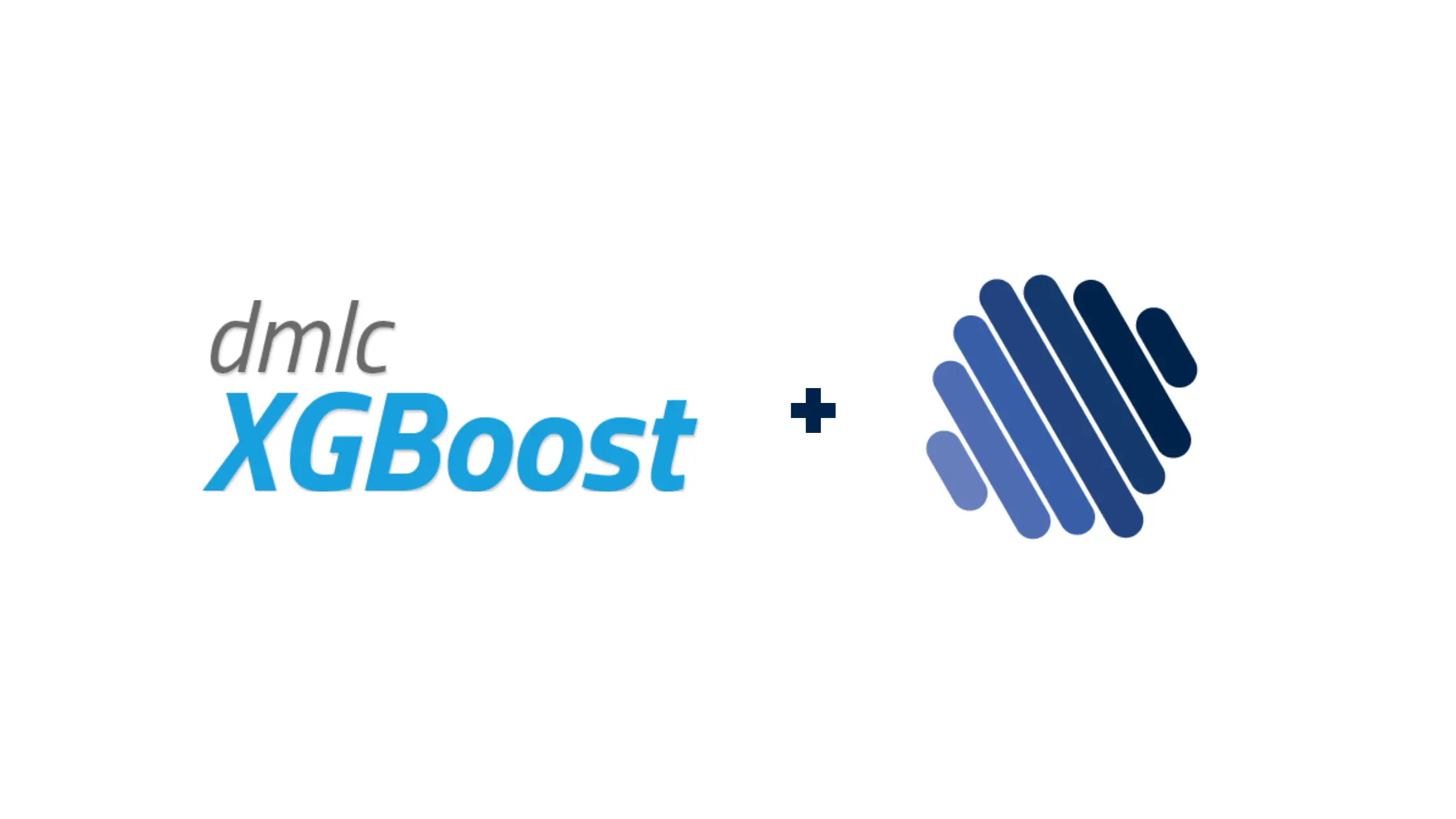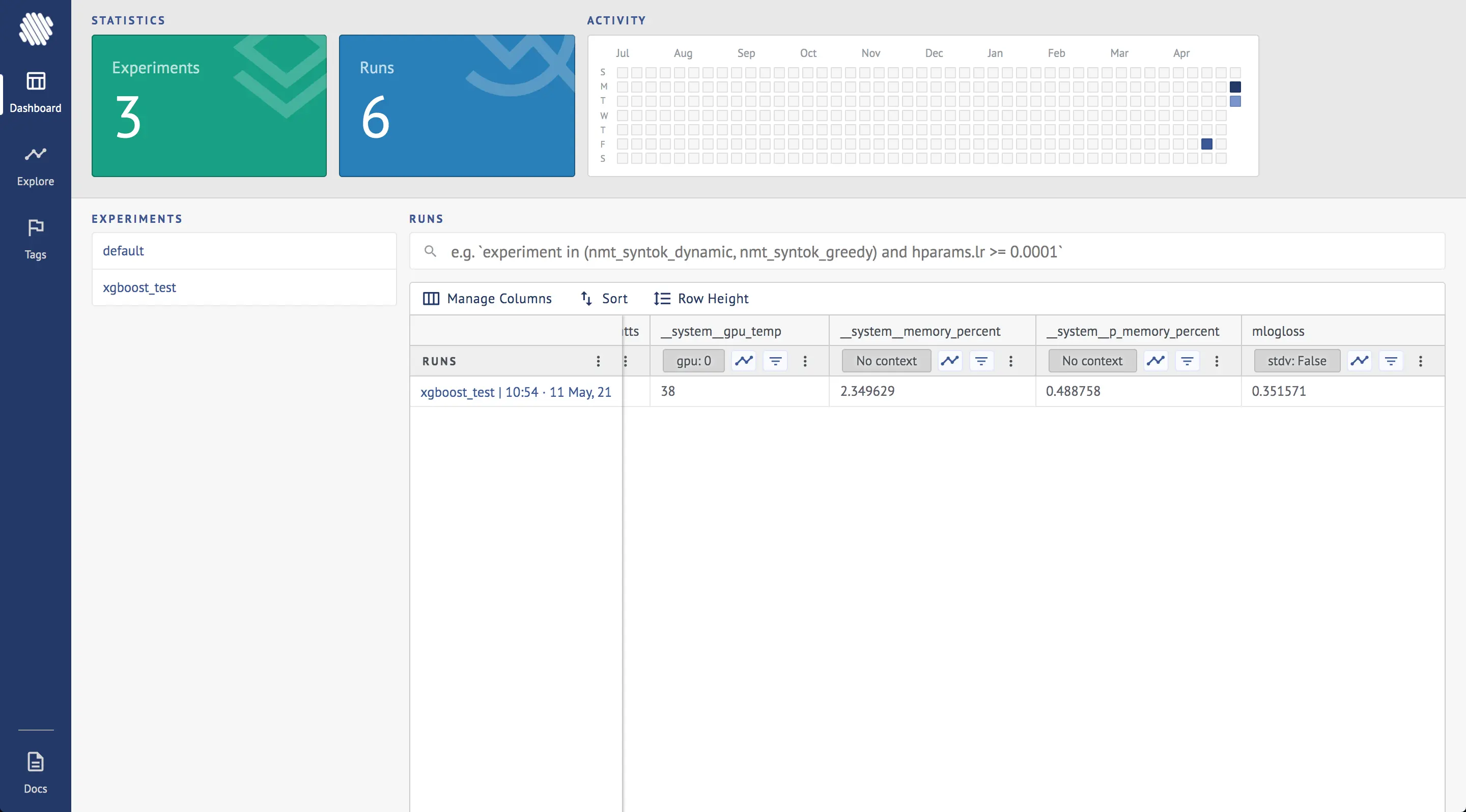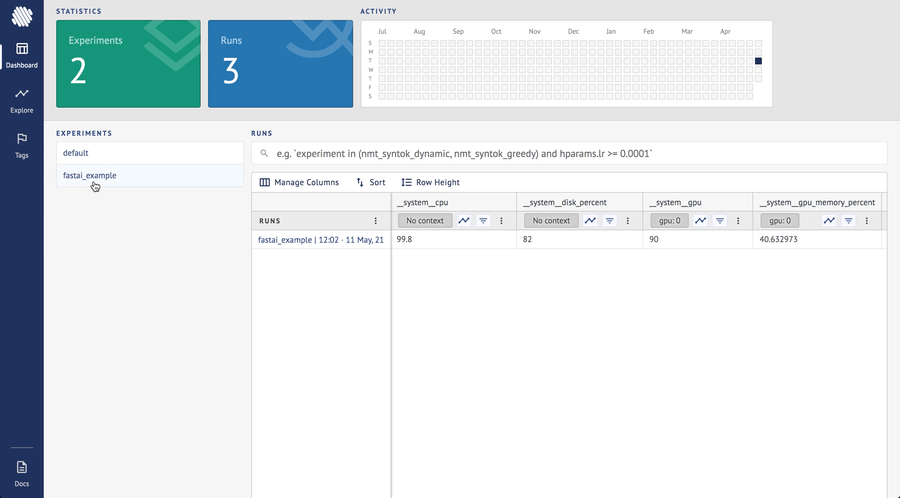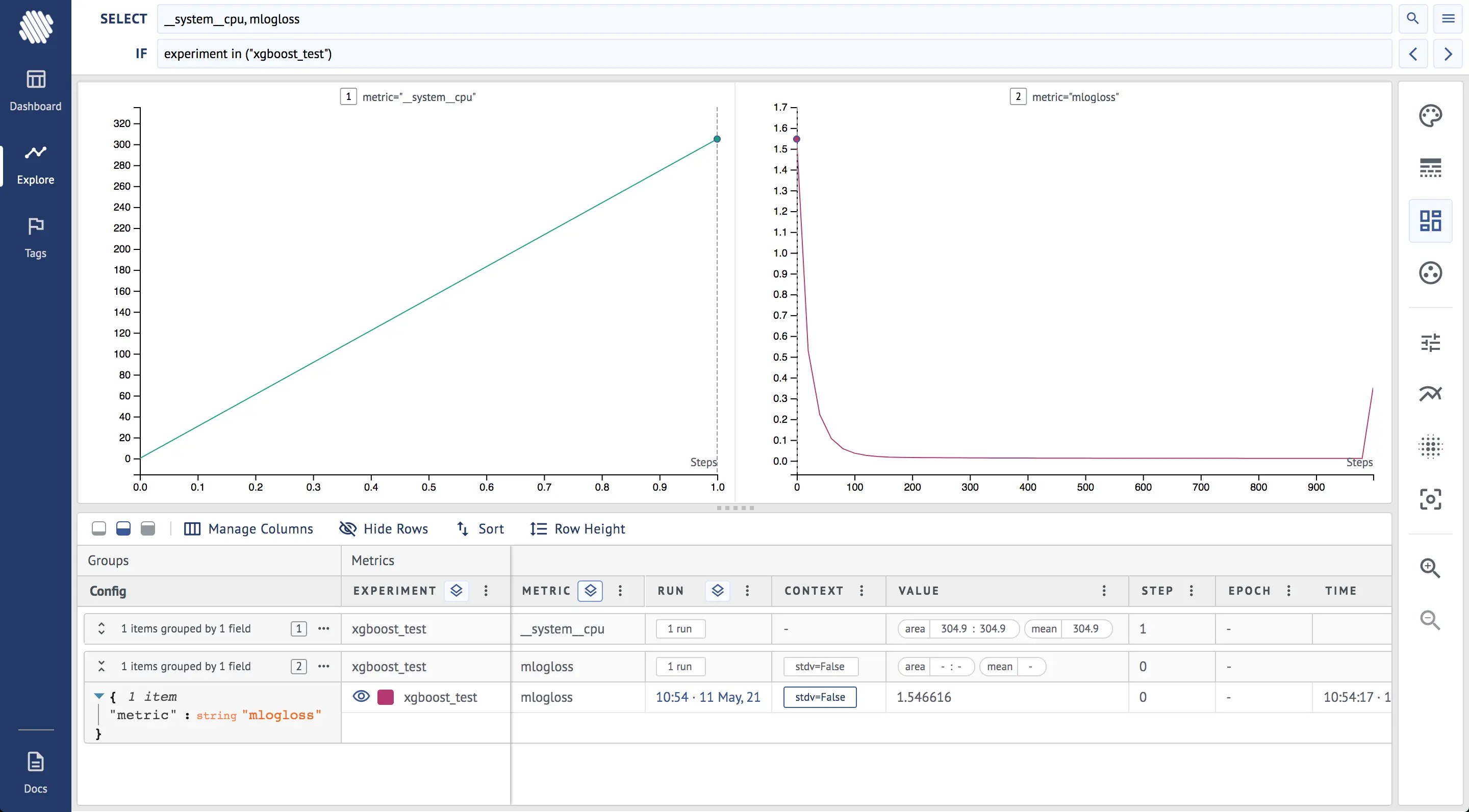May 17, 2021

# An end-to-end example of Aim logger used with XGBoost library

Author:## What is Aim?

Aim is an open-source tool for AI experiment comparison. With more resources and complex models, more experiments are ran than ever. You can indeed use Aim to deeply inspect thousands of hyperparameter-sensitive training runs.

## What is XGBoost?

XGBoost is an optimized gradient boosting library with highly  efficient,  flexible,  and  portable design. XGBoost provides a parallel tree boosting (also known as GBDT, GBM) that solves many data science problems in a fast and accurate way. Subsequently, the same code runs on major distributed environment (Kubernetes, Hadoop, SGE, MPI, Dask) and can solve problems beyond billions of examples.

## How to use Aim with XGBoost?

Check out end-to-end Aim integration examples with multiple frameworks here. In this tutorial, we are going to show how to integrate Aim and use AimCallback in your XGBoost code.

``````# You should download and extract the data beforehand. Simply by doing this:
# wget https://archive.ics.uci.edu/ml/machine-learning-databases/dermatology/dermatology.data

from __future__ import division

import numpy as np
import xgboost as xgb
from aim.xgboost import AimCallback

# label need to be 0 to num_class -1
converters={33: lambda x:int(x == '?'), 34: lambda x:int(x) - 1})
sz = data.shape

train = data[:int(sz * 0.7), :]
test = data[int(sz * 0.7):, :]

train_X = train[:, :33]
train_Y = train[:, 34]

test_X = test[:, :33]
test_Y = test[:, 34]
print(len(train_X))

xg_train = xgb.DMatrix(train_X, label=train_Y)
xg_test = xgb.DMatrix(test_X, label=test_Y)
# setup parameters for xgboost
param = {}
# use softmax multi-class classification
param['objective'] = 'multi:softmax'
# scale weight of positive examples
param['eta'] = 0.1
param['max_depth'] = 6
param['num_class'] = 6

watchlist = [(xg_train, 'train'), (xg_test, 'test')]
num_round = 50
bst = xgb.train(param, xg_train, num_round, watchlist)
# get prediction
pred = bst.predict(xg_test)
error_rate = np.sum(pred != test_Y) / test_Y.shape
print('Test error using softmax = {}'.format(error_rate))

# do the same thing again, but output probabilities
param['objective'] = 'multi:softprob'
bst = xgb.train(param, xg_train, num_round, watchlist,
callbacks=[AimCallback(repo='.', experiment='xgboost_test')])
# Note: this convention has been changed since xgboost-unity
# get prediction, this is in 1D array, need reshape to (ndata, nclass)
pred_prob = bst.predict(xg_test).reshape(test_Y.shape, 6)
pred_label = np.argmax(pred_prob, axis=1)
error_rate = np.sum(pred_label != test_Y) / test_Y.shape
print('Test error using softprob = {}'.format(error_rate))``````

As you can see on line 49, AimCallback is imported from `aim.xgboost` and passed to `xgb.train` as one of the callbacks. Aim session can open and close by the AimCallback and the metrics and hparamsstore by XGBoost. In addition to that, thesystem measures pass to Aim as well.

## What it looks like?

After you run the experiment and the `aim up` command in the `aim_logs`directory, Aim UI will be running. When first opened, the dashboard page will come up.To explore the run, we should:

• Choose the `xgboost_test`experiment.
• Select the metrics to explore.
• Divide into charts by metrics.

For example, the gif below illustrates the steps above.\ So this is what the final result looks like.As easy as that, we can analyze the runs and the system usage.